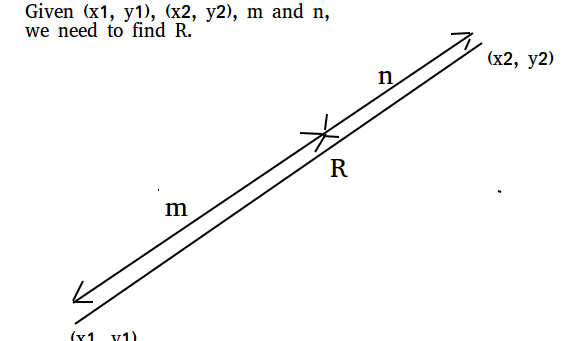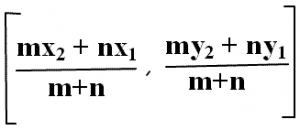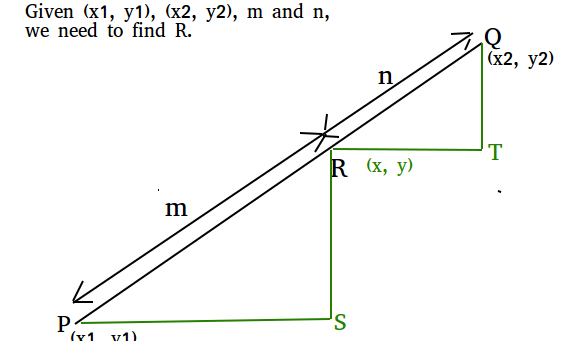# Section formula (Point that divides a line in given ratio)

Given two coordinates (x1, y1) and (x2, y2), and m and n, find the co-ordinates that divides that divides the line joining (x1, y1) and (x2, y2) in the ratio m : nExamples:

```Input : x1 = 1, y1 = 0, x2 = 2 y2 = 5,
m = 1, n = 1
Output : (1.5, 2.5)
Explanation: co-ordinates (1.5, 2.5)
divides the line in ratio 1 : 1

Input : x1 = 2, y1 = 4, x2 = 4, y2 = 6,
m = 2, n = 3
Output : (2.8, 4.8)
Explanation: (2.8, 4.8) divides the line
in the ratio 2:3
```

## Recommended: Please try your approach on {IDE} first, before moving on to the solution.

The section formula tells us the coordinates of the point which divides a given line segment into two parts such that their lengths are in the ratio m : n## C++

 `// CPP program to find point that divides ` `// given line in given ratio. ` `#include ` `using` `namespace` `std; ` ` `  `// Function to find the section of the line ` `void` `section(``double` `x1, ``double` `x2, ``double` `y1, ` `              ``double` `y2, ``double` `m, ``double` `n) ` `{ ` `    ``// Applying section formula ` `    ``double` `x = ((n * x1) + (m * x2)) / ` `                            ``(m + n); ` `    ``double` `y = ((n * y1) + (m * y2)) / ` `                             ``(m + n); ` ` `  `    ``// Printing result ` `    ``cout << ``"("` `<< x << ``", "``; ` `    ``cout << y << ``")"` `<< endl; ` `} ` ` `  `// Driver code ` `int` `main() ` `{ ` `    ``double` `x1 = 2, x2 = 4, y1 = 4, ` `           ``y2 = 6, m = 2, n = 3; ` `    ``section(x1, x2, y1, y2, m, n); ` `    ``return` `0; ` `} `

## Java

 `// Java program to find point that divides ` `// given line in given ratio. ` `import` `java.io.*; ` ` `  `class` `sections { ` `    ``static` `void` `section(``double` `x1, ``double` `x2, ` `                        ``double` `y1, ``double` `y2, ` `                        ``double` `m, ``double` `n) ` `    ``{ ` `        ``// Applying section formula ` `        ``double` `x = ((n * x1) + (m * x2)) / ` `                    ``(m + n); ` `        ``double` `y = ((n * y1) + (m * y2)) / ` `                    ``(m + n); ` ` `  ` `  `        ``// Printing result ` `        ``System.out.println(``"("` `+ x + ``", "` `+ y + ``")"``); ` `    ``} ` ` `  `    ``public` `static` `void` `main(String[] args) ` `    ``{ ` `        ``double` `x1 = ``2``, x2 = ``4``, y1 = ``4``, ` `               ``y2 = ``6``, m = ``2``, n = ``3``; ` `        ``section(x1, x2, y1, y2, m, n); ` `    ``} ` `} `

## Python

 `# Python program to find point that divides ` `# given line in given ratio. ` `def` `section(x1, x2, y1, y2, m, n): ` ` `  `    ``# Applying section formula ` `    ``x ``=` `(``float``)((n ``*` `x1)``+``(m ``*` `x2))``/``(m ``+` `n) ` `    ``y ``=` `(``float``)((n ``*` `y1)``+``(m ``*` `y2))``/``(m ``+` `n) ` ` `  `    ``# Printing result ` `    ``print` `(x, y) ` ` `  `x1 ``=` `2` `x2 ``=` `4` `y1 ``=` `4` `y2 ``=` `6` `m ``=` `2` `n ``=` `3` `section(x1, x2, y1, y2, m, n) `

## C#

 `// C# program to find point that divides ` `// given line in given ratio. ` `using` `System; ` ` `  `class` `GFG { ` `     `  `    ``static` `void` `section(``double` `x1, ``double` `x2, ` `                        ``double` `y1, ``double` `y2, ` `                          ``double` `m, ``double` `n) ` `    ``{ ` `         `  `        ``// Applying section formula ` `        ``double` `x = ((n * x1) + (m * x2)) / ` `                                    ``(m + n); ` `                                     `  `        ``double` `y = ((n * y1) + (m * y2)) / ` `                                   ``(m + n); ` ` `  `        ``// Printing result ` `        ``Console.WriteLine(``"("` `+ x + ``", "` `+ y + ``")"``); ` `    ``} ` ` `  `    ``// Driver code ` `    ``public` `static` `void` `Main() ` `    ``{ ` `         `  `        ``double` `x1 = 2, x2 = 4, y1 = 4, ` `                ``y2 = 6, m = 2, n = 3; ` `                 `  `        ``section(x1, x2, y1, y2, m, n); ` `    ``} ` `} ` ` `  `// This code is contributed by vt_m. `

## PHP

 ` `

Output:

```(2.8, 4.8)
```

How does this work?```From our diagram, we can see,
PS = x – x1 and RT = x2 – x

We are given,

PR/QR = m/n

Using similarity, we can write
RS/QT = PS/RT = PR/QR

Therefore, we can write
PS/RR = m/n
(x - x1) / (x2 - x) = m/n

From above, we get
x = (mx2 + nx1) / (m + n)

Similarly, we can solve for y.```

My Personal Notes arrow_drop_upCheck out this Author's contributed articles.

If you like GeeksforGeeks and would like to contribute, you can also write an article using contribute.geeksforgeeks.org or mail your article to contribute@geeksforgeeks.org. See your article appearing on the GeeksforGeeks main page and help other Geeks.

Please Improve this article if you find anything incorrect by clicking on the "Improve Article" button below.

Improved By : jit_t